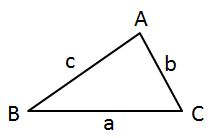# SAT Subject Test Mathematics Level 2: Tutoring Solution Final Exam

Free Practice Test Instructions:

Choose your answer to the question and click 'Continue' to see how you did. Then click 'Next Question' to answer the next question. When you have completed the free practice test, click 'View Results' to see your results. Good luck!

#### Question 6 6. What is the domain of the function below?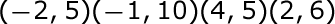#### Question 7 7. Solve the Combination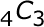#### Question 8 8. What kind of relationship do you see on this graph?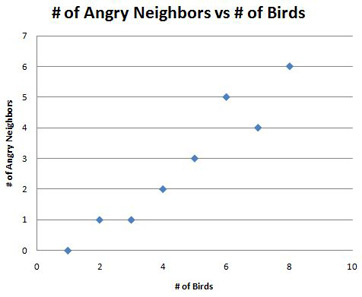#### Question 10 10. Find the roots of the equation.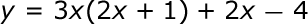#### Question 13 13. Add the following rational expressions.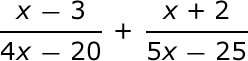#### Question 14 14. In the pictured triangle, side b is 12 and side c is 16. If ∠A is 84 degrees, then approximately how long is side a? Round the value of the cosine to the nearest whole number.